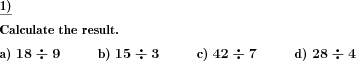Custom math worksheets at your fingertipsDetails for problem "Division times table 1-10"

Quickname: 1717

Elementary School, Primary School.

Summary

Division problems from the multiplication tables

ExampleDescription

Division exercises are set from the 1x to 10x multiplication tables. The dividend is a product of the multiplication tables. The divisor can be chosen in the range from one to ten or can be determined randomly for each problem.

All quotients are natural numbers without remainder or decimal places.

The number of problems can be chosen.

This exercise is suitable to consolidate the knowledge of the times tables from one to ten. While the multiplication tasks from the multiplication tables support the learning of the rows of the tables from left to right, these division tasks allow to practice the rows from left to right, i.e. to infer from the product to the factors. This type of task is therefore an important and essential part of a complete memorization of the lower multiplication tables.

Download free printable worksheets for this math problem here. The worksheet contains the problems only, the solution sheet includes the answers. Just click on the respective link.

•Worksheet 1Solution sheet with answers
•Worksheet 2Solution sheet with answers
•Worksheet 3Solution sheet with answers

If you can not see the solution sheets for download, they may be filtered out by an ad blocker that you may have installed. If this is the case, please allow ads for this page and reload the page. The solution sheets will then reappear.

• Do these sample worksheets do not really fit?
• Do you need more math worksheets, with a different level of difficulty?
• Would you like to combine different problems on a worksheet and adjust them to your needs?
• As a teacher, you can put together your own worksheets using the automatically generated math problems provided.
With a free initial credit, you can start creating your own math worksheets in a few minutes.

You can try it for free! Register here, to create custom worksheets now!

Customization options for this problem

Parameter
Possible values
Number of problems
1, 2, 3, 4, 5, 6, 7, 8, 9, 10
Number to train
all, 1, 2, 3, 4, 5, 6, 7, 8, 9, 10

Similar problems

Remark
Description
As a series of quotients with factors of 10s
Division problem series with divisor and dividend alternating with factor ten.
As division using place values with larger numbers
Divide by splitting the dividend.
Generic division problem
Division of two whole numbers, with remainder, written long division method illustrated.
Is also available as a fill-in task
Simple times table problems where the product and a factor are given.Deutsche Version dieser Aufgabe
These informational pages with samples describe math problems that can be combined on custom math worksheets with solutions for home and K-12 school use.
Deutsche Seiten
×